# The Midpoint Theorem and Formula

Once a while in mathematics, we really need to find the midpoint between two other points, that is, the point that is exactly in the middle of the two other points. A good example is if you have to find the point at which a line bisects or divides a given line segment into two equal parts. The midpoint formula is quite simple and you should endeavour to know how to derive it for future use.

The midpoint formula can be conceived in terms of finding the middle number that exists between two given numbers such as 10 and 15. By adding the two numbers and dividing by 2, we obtain the exact middle number as follows: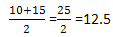Exactly the same way, the midpoint formula works. Let us consider the following question:

Find the midpoint between the points (-2, 4) and (6,-12).

Solution:

If we apply the midpoint formula, we have the following: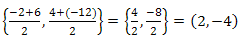Hence, the coordinates of the midpoint = (2,-4)

The Midpoint Formula:

The midpoint formula is, therefore, given as: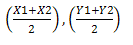This formula can be applied for solving different questions such as the followings:

1. If a line segment has its endpoints given as (-1.8, 3.9) and (8.2,-1.1), determine whether the equation y=2x-4.9 is a bisector of this line segment.

Solution:

The exact midpoint of the line segment can only be determined by applying the midpoint formula and not by graphical method. Hence, by applying the midpoint formula, we have: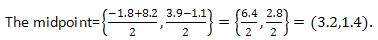Now, check to see if this point is on the line y=2x-4.9.

y=2x-4.9

y=2(3.2)-4.9=6.4-4.9=1.5. But, y, ought to be equal to 1.4 as the y-coordinate of the midpoint indicates. Therefore, y=2x-4.9 is not a bisector of the line segment.

2. Find the bisector of the line segment given above.

To find the perpendicular bisector of this line segment, you will need to solve the problem in a multi-parts manner. Note that this is one of the typical questions you will surely come across as you proceed in your learning of maths particularly the straight lines. The procedure for solving this problem is simple and straight forward and it is given as follows:

First of all, determine the midpoint of the line segment by applying the midpoint formula given above.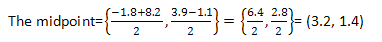The slope of the line segment is needed here; therefore, we obtain the slope as follows:

Slope,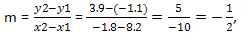this is a negative reciprocal which must be flipped.

So, the slope, m=2 (since negative reciprocal means that it should be changed).

The equation of the bisector of the line segment is therefore obtained as follows:

y – y1 = slope(x – x1)

y – 1.4 = 2(x – 3.2)

y = 2x – 5.

0 Shares
Filed under Math.